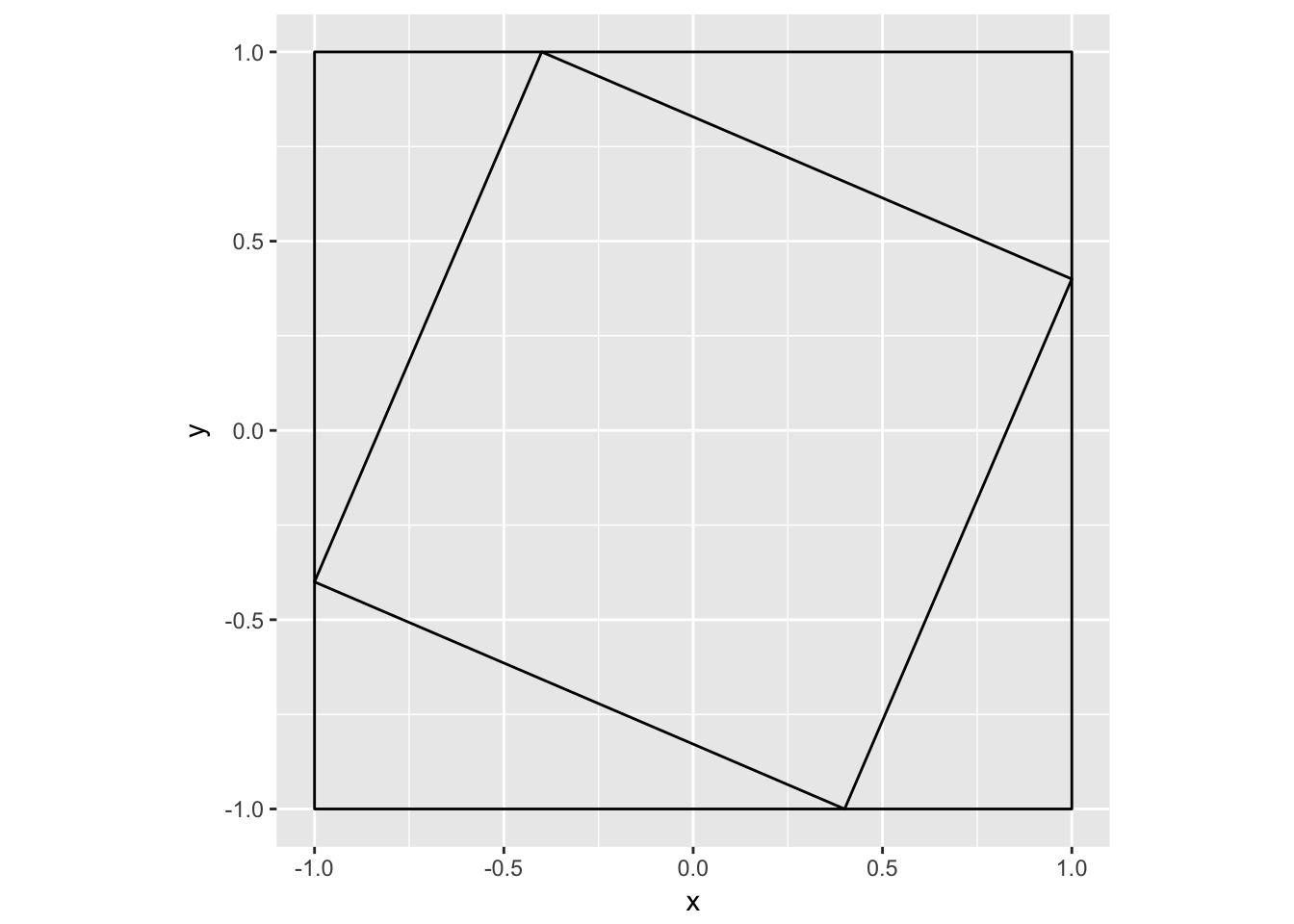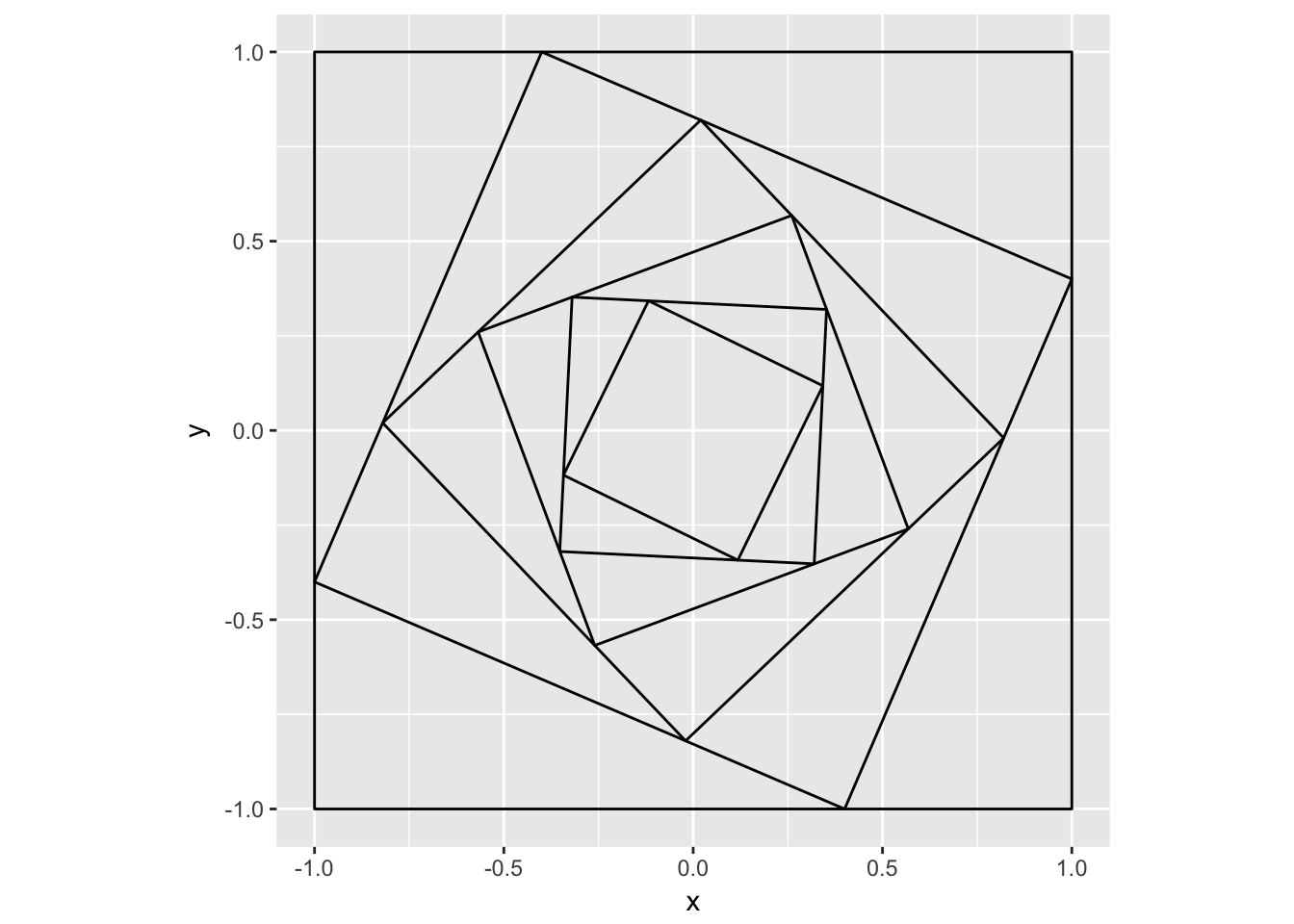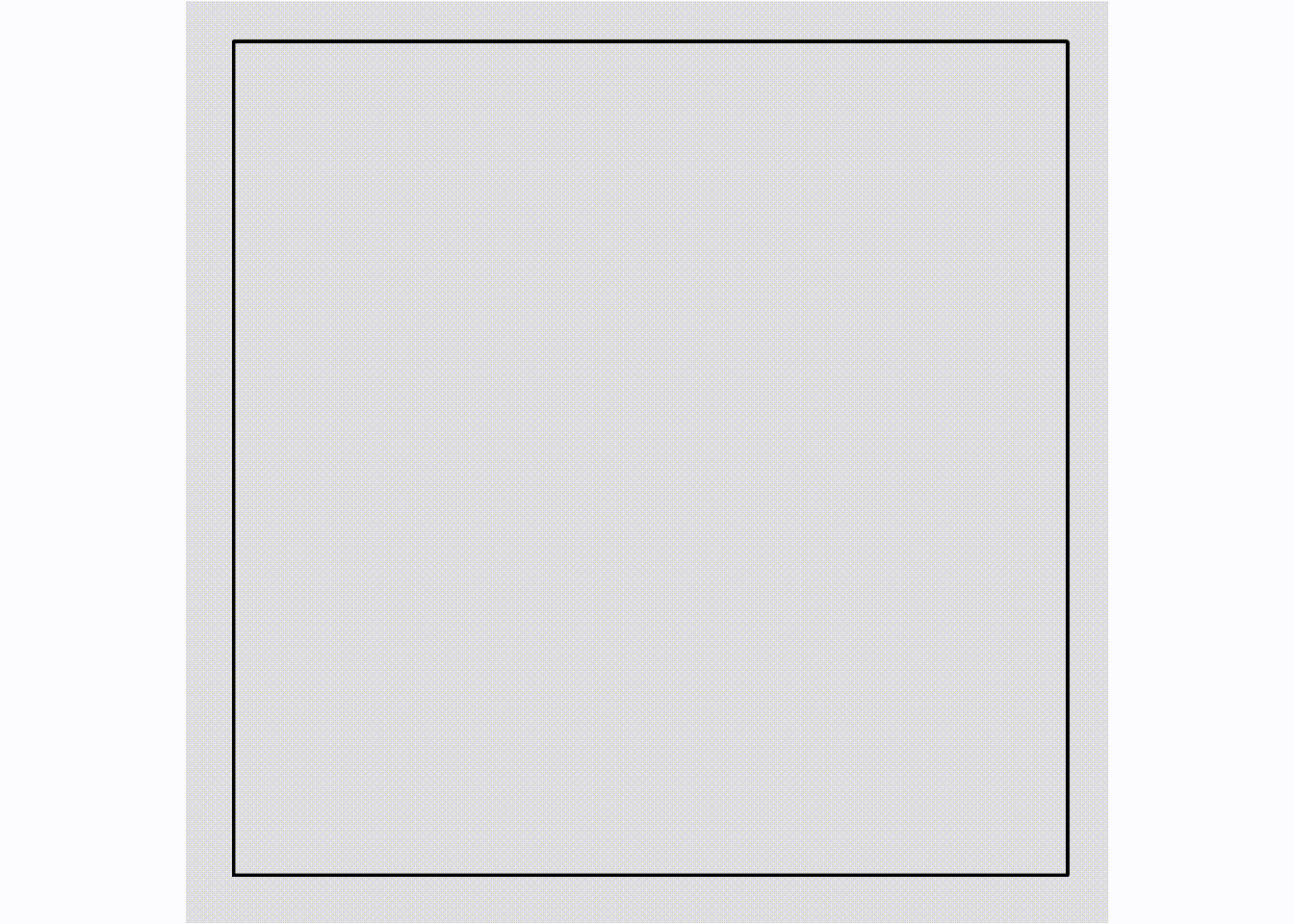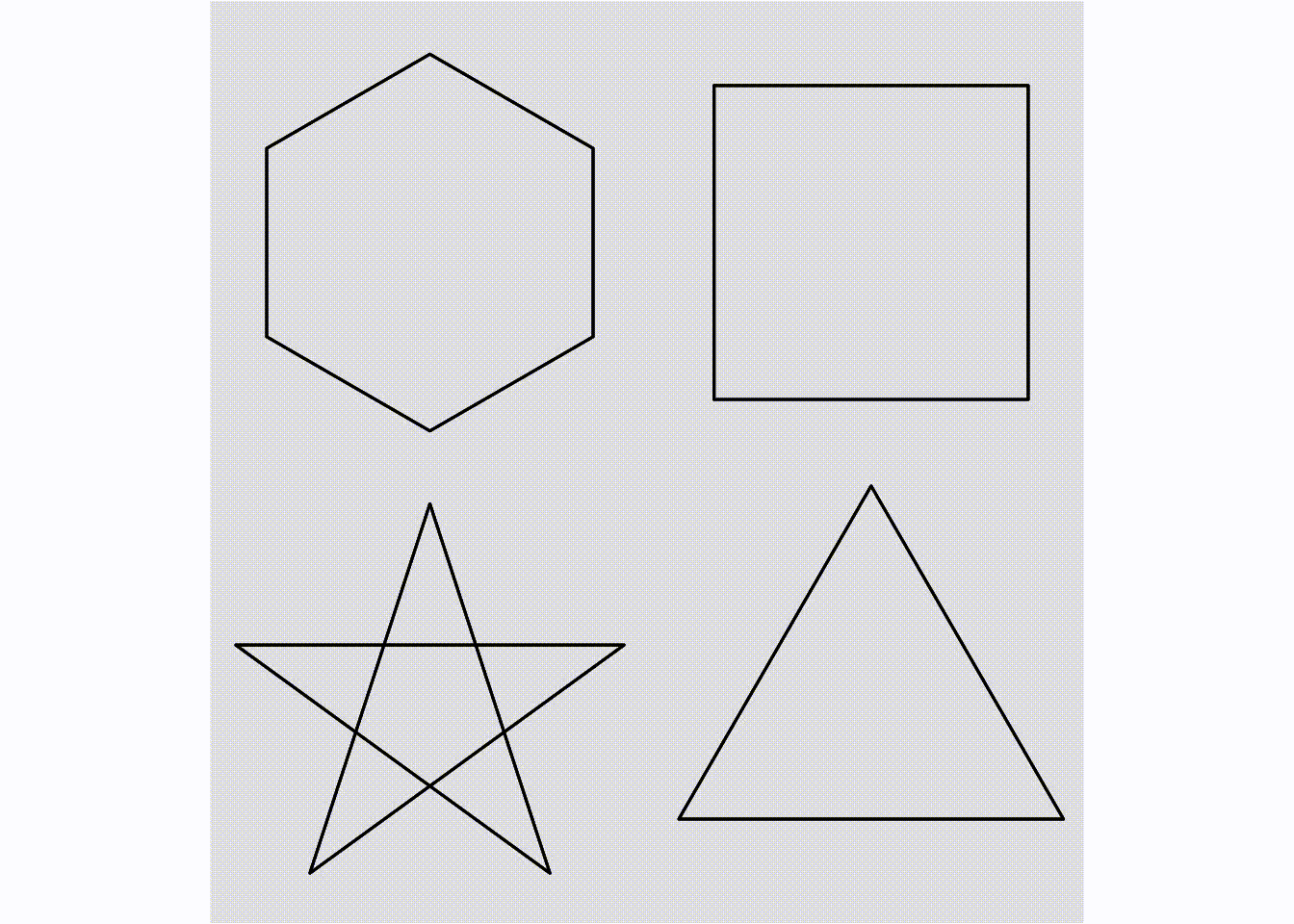# Creating a Bees and Bombs gif in R

## Oct 20, 2016 · 1282 words · 7 minutes read R design

I love the work of @beesandbombs and while some of his creations are a poor fit for redoing in R, one of his latest got me wondering how I would recreate it in the venerable statistics language…

The gif in question can be seen here (I don’t know to make those fancy tweet cards using jekyll and GitHub pages).

Update: I now know how to embed tweets 😄

While it would be nice if this was something easily solvable using tweenr it is unfortunately not, as there’s no direct path between any of the states in the animation. Each square relies on the orientation of the bounding square and so the bounding sqaure must be calculated before we can know how the inner square will move, but since the bounding sqaure moves as well we’ll need to do this for each frame.

Fortunately, if you look closely on the animation you can realise that the only calculation happening is a linear interpolation between two points. The corner of an inner square simply traces the path between two corners of the bounding square. So, for each side we could define a simple linear function and solve it for the given position…

But wait, that means creating four linear functions for each square - with 16 squares and a framerate of 15 frames/sec this will result in 3840 functions that needs to be created for a 4 sec animation. Seems like overkill…

Fortunately we can come to another realization: If we consider each side a vector we are simply multiplying the progression of the animation onto the vector to get the corner positions of the inner square. So, given a square:

``````sq <- data.frame(
x = c(-1, 1, 1, -1),
y = c(1, 1, -1, -1)
)``````

We can calculate the corners of the inner square at time t (between 0 and 1) like so:

``````sq_to_vec <- function(square) {
data.frame(
x = square\$x[c(2,3,4,1)] - square\$x,
y = square\$y[c(2,3,4,1)] - square\$y
)
}
vec_mult <- function(vec, t) {
vec\$x <- vec\$x * t
vec\$y <- vec\$y * t
vec
}
vec_repos <- function(vec, square) {
square\$x <- square\$x + vec\$x
square\$y <- square\$y + vec\$y
square
}
calc_inner <- function(square, t) {
square %>%
sq_to_vec() %>%
vec_mult(t) %>%
vec_repos(square)
}``````

I’ve probably gone overboard with the code refactoring, but this is mainly to illustrate what’s going on. Let’s try it out:

``````sq1 <- calc_inner(sq, 0.3)
sq_dat <- rbind(sq, sq1)
sq_dat\$group <- rep(1:2, each = 4)
ggplot(sq_dat) +
geom_polygon(aes(x, y, group = group), fill = NA, colour = 'black') +
coord_fixed()``````that seems right…

The next task is to do this for multiple squares within each others. True R users would quickly think that some `*apply()` function can solve this for us but, alas, each computation relies on the result of the prior. Then what? Are we force into using a for-loop in this day an age where functional programming is all the rage? Fear not! `Reduce()` is here to save you…

``````calc_frame <- function(start, t, n) {
squares <- rep(t, n)
squares <- Reduce(function(sq1, t) {
append(sq1, list(calc_inner(sq1[[length(sq1)]], t)))
}, squares, init = list(start))
squares <- do.call(rbind, squares)
squares\$group <- rep(seq_len(n + 1), each = 4)
squares
}
squares <- calc_frame(sq, 0.3, 5)
ggplot(squares) +
geom_polygon(aes(x, y, group = group), fill = NA, colour = 'black') +
coord_fixed()``````Reduce can be a really powerfull way of abstracting away computations relying on the prior result…

Now all that is left is to call the `calc_frame()` function for a number of frames that covers t = 0 – 1

``````frame_t <- seq(0, 1, length.out = 100)
squares <- lapply(seq_along(frame_t), function(frame) {
squares <- calc_frame(sq, frame_t[frame], 16)
squares\$frame <- frame
squares
})
squares <- do.call(rbind, rev(squares))
p <- ggplot(squares) +
geom_polygon(aes(x, y, group = group, frame = frame), fill = NA, colour = 'black') +
coord_fixed() +
theme_void() +
theme(plot.background = element_rect(fill = 'grey90'))
gganimate(p, title_frame = FALSE)``````Now we could definetly call it a day now as we have reached our objective, but I want to talk about one last thing before I wrap up: Code generalization. We set out to recreate the Bees and Bombs animation so we were completely focused on making this work for squares. While our code would work nicely for rectangles as well it doesn’t generalize to other polygons. There is no need for this constraint though. Nothing in our setup should make this specific for 4-sided objects so with a little care towards generalization we can improve our code and make it generally applicable. Fortunately it is not a big change. The only function that makes any assumptions about the shape of our polygon is the `sq_to_vec()` function. Some of the other function arguments imply a square though so we’ll rewrite these as well:

``````# Don't assume a shape with four corners
shape_to_vec <- function(shape) {
data.frame(
x = c(shape\$x[-1], shape\$x) - shape\$x,
y = c(shape\$y[-1], shape\$y) - shape\$y
)
}
# Rename square to shape
vec_repos <- function(vec, shape) {
shape\$x <- shape\$x + vec\$x
shape\$y <- shape\$y + vec\$y
shape
}
calc_inner <- function(shape, t) {
shape %>%
shape_to_vec() %>%
vec_mult(t) %>%
vec_repos(shape)
}
calc_frame <- function(start, t, n) {
shapes <- rep(t, n)
shapes <- Reduce(function(sq1, t) {
append(sq1, list(calc_inner(sq1[[length(sq1)]], t)))
}, shapes, init = list(start))
shapes <- do.call(rbind, shapes)
shapes\$group <- rep(seq_len(n + 1), each = nrow(start))
shapes
}``````

That was easy - let’s try to make something crazy with this:

``````trans <- radial_trans(c(0, 1), c(0, 1), pad = 0)
triangle <- trans\$transform(rep(sqrt(2), 4), seq(0, 1, length.out = 4))[1:3, ]
triangles <- lapply(seq_along(frame_t), function(frame) {
triangles <- calc_frame(triangle, frame_t[frame], 16)
triangles\$frame <- frame
triangles
})
triangles <- do.call(rbind, rev(triangles))
triangles\$shape <- 'triangle'
star <- trans\$transform(rep(1.3, 6), seq(0, 1, length.out = 6))[c(1, 3, 5, 2, 4), ]
stars <- lapply(seq_along(frame_t), function(frame) {
stars <- calc_frame(star, frame_t[frame], 16)
stars\$frame <- frame
stars
})
stars <- do.call(rbind, rev(stars))
stars\$shape <- 'star'
hexagon <- trans\$transform(rep(1.2, 7), seq(0, 1, length.out = 7))[1:6, ]
hexagons <- lapply(seq_along(frame_t), function(frame) {
hexagons <- calc_frame(hexagon, frame_t[frame], 16)
hexagons\$frame <- frame
hexagons
})
hexagons <- do.call(rbind, rev(hexagons))
hexagons\$shape <- 'hexagon'
squares\$shape <- 'square'
shapes <- rbind(squares, stars, triangles, hexagons)
p <- ggplot(shapes) +
geom_polygon(aes(x, y, group = -group, frame = frame, colour = group),
fill = NA) +
scale_color_gradient(low = 'black', high = 'grey90', trans = 'log') +
facet_wrap(~shape) +
coord_fixed() +
theme_void() +
theme(plot.background = element_rect(fill = 'grey90'),
legend.position = 'none',
strip.text = element_blank())
gganimate(p, title_frame = FALSE)``````As generalizations go, this is pretty good. While all of the above is quite simple I hope it serves to illustrate how you can think about the code you write and how small changes can make it broader applicable. Even if there is no way that the code should be applied in any other context than the one at hand, thinking about generalization helps you identify the hidden assumptions in your code, so it is never a wasted exercise.

Another point I hope I have made is how animations are often really simple. A lot of R users are accustumed to static plots and find animations to be very daunting. In reality it is often just a matter of identifying how key points travel around as time pass and everything else will sort itself out. What I admire about Bees and Bombs is how he can take seemingly simple relationships between shapes and transformations and mix it together in a way that makes the whole greater than the sums of the parts. I invite anyone who wish to improve their understanding of animations in R to recreate one of his pieces - it might be simpler than you think…